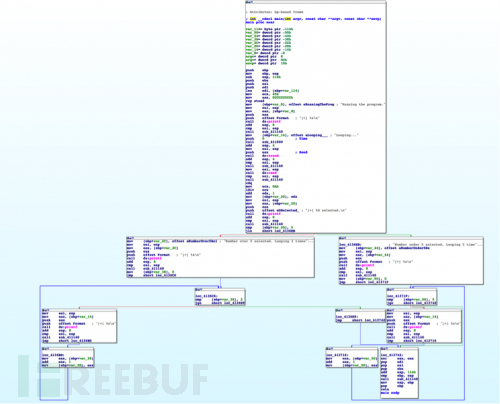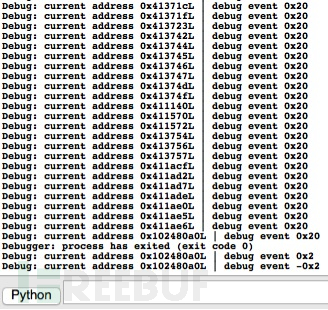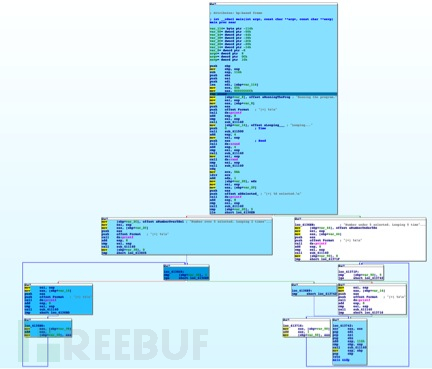# IDApython教程（四）

#include "stdafx.h"
#include <stdlib.h>
#include <time.h>

int _tmain(int argc, _TCHAR* argv[])
{
char* start = "Running the program.";
printf("[+] %s\n", start);
char* loop_string = "Looping...";

srand (time(NULL));
int bool_value = rand() % 10 + 1;
printf("[+] %d selected.\n", bool_value);
if(bool_value > 5)
{
char* over_five = "Number over 5 selected. Looping 2 times.";
printf("[+] %s\n", over_five);
for(int x = 0; x < 2; x++)
printf("[+] %s\n", loop_string);
}
else
{
char* under_five = "Number under 5 selected. Looping 5 times.";
printf("[+] %s\n", under_five);
for(int x = 0; x < 5; x++)
printf("[+] %s\n", loop_string);
}
return 0;
}RunTo(BeginEA())
event = GetDebuggerEvent(WFNE_SUSP, -1)

EnableTracing(TRACE_STEP, 1)
event = GetDebuggerEvent(WFNE_ANY|WFNE_CONT, -1)

while True:
event = GetDebuggerEvent(WFNE_ANY, -1)
if event <= 1: breakdef get_new_color(current_color):
colors = [0xffe699, 0xffcc33, 0xe6ac00, 0xb38600]
if current_color == 0xFFFFFF:
return colors
if current_color in colors:
pos = colors.index(current_color)
if pos == len(colors)-1:
return colors[pos]
else:
return colors[pos+1]
return 0xFFFFFF

new_color = get_new_color(current_color)
SetColor(addr, CIC_ITEM, new_color)heads = Heads(SegStart(ScreenEA()), SegEnd(ScreenEA()))
SetColor(i, CIC_ITEM, 0xFFFFFF)

heads = Heads(SegStart(ScreenEA()), SegEnd(ScreenEA()))
SetColor(i, CIC_ITEM, 0xFFFFFF)

def get_new_color(current_color):
colors = [0xffe699, 0xffcc33, 0xe6ac00, 0xb38600]
if current_color == 0xFFFFFF:
return colors
if current_color in colors:
pos = colors.index(current_color)
if pos == len(colors)-1:
return colors[pos]
else:
return colors[pos+1]
return 0xFFFFFF

RunTo(BeginEA())
event = GetDebuggerEvent(WFNE_SUSP, -1)

EnableTracing(TRACE_STEP, 1)
event = GetDebuggerEvent(WFNE_ANY|WFNE_CONT, -1)
while True:
event = GetDebuggerEvent(WFNE_ANY, -1)
new_color = get_new_color(current_color)
if event <= 1: break